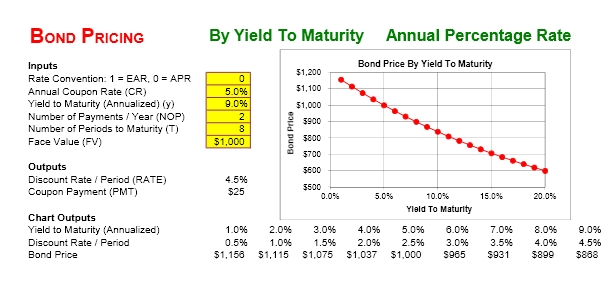# Bond Yield to Maturity Calculator

Exceltemplates.org – For investors, Bond Yield to Maturity Calculator is an important tool which can assist them in calculating their earnings once they have bought today’s bond. This calculator can be made by putting on the required data and formulas manually in Microsoft Excel worksheets; or if you want to make it simpler, you can go online and grab it fast and more easily.## Bond Yield to Maturity Calculator Definition

Before using Bond YTM calculator, you have to first its definition. Bond Yield to Maturity Calculator simply refers to the return rate investors will get when they buy a bond within the most updated market price, and hold it up to maturity. Meanwhile, YTM (Yield to Maturity) is generally defined as an index used to measure the bond’s attractiveness. Bond Yield to Maturity Calculator is then used by using assumptions that the bond is going to be held up to its maturity, while all of the principal payments and coupons will be on schedule. By using this calculator, the investors will be able to estimate how much benefit they can expect from the bonds they have already got.

## How to Use Bond Yield to Maturity Calculator

• There are things you should calculate to get the final result of Bond Yield to Maturity Calculator, such as the following:
• Subtract the bond purchase price from its par value (you will get the discount)
• Use bond’s year to maturity to divide the result of first formula (discount); resulted in annual gain of capital
• Add yearly interest to capital gain to get the annual return
• The first yield can be found out by dividing annual return by bond’s purchase price
• Subtract the  par value by annual capital gain; then divide annual return by its formula’s result to get the second yield
• Average both of the yields to get the YTM
Check this template >  Rental Invoice Template

There are plenty of excel calculator you can find and this one is one of them.Using Microsoft Excel to simplify your spreadsheet tasks is not as difficult as you think. I learned how to use it in less than 24 hours and rely on internet to find the solution if I got stuck. I am not an Excel expert, but here are all useful spreadsheets I have collected and created.